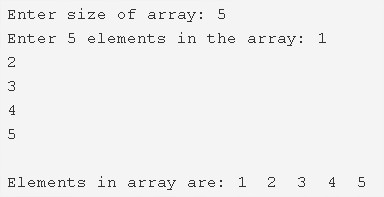# C Program To Read & Print Elements Of Array | C Programs

C Program to read and print elements of an array – In this distinct article, we will detail in on the various ways to read and print the elements of an array in C programming.

Suitable examples and sample programs have also been added so that you can understand the whole thing very clearly. The compiler has also been added with which you can execute it yourself for read and print elements of array.

The methods discussed in the current piece are as follows:

• Using Standard Method
• Using Function
• Using Recursion

An array is a collection or a sequential order to various elements, with or without a particular order. Arrays usually consist of information that is stored at respective pointers in C programming.As you can see, in this array, the size of the array is defined at first.

The size of this array is 5.

After that, the elements are entered accordingly for the array.

The elements entered in this array are as follows:

1, 2, 3, 4 and 5.

Hence, after it is given a command to print the elements, all the five elements will be printed as per the order they were entered into the array.

Thus, the different means of achieving the same in C programming is as follows:

## Using Standard Method

1. Read the size of an array and store that value into the variable n.

2) Scanf() function reads the entered element and initialize that element to a[i] until all iterations of for loop as scanf(“%d”,&a[i]) using for loop for(i=0;i<n;i++).

3) Print the array elements of a[] using for loop which iterates i=0 to i<size of an array.

printf(“%d”,a[i]),it prints the elements of an array from i=0 to i<n using for loop.

Output:

### Using Function – Read & Print an element in Array

1. Set of code which performs a task is called a function.

2) We have two functions in this program those are input(),output().

3) The function input() performs read operation, which reads entered elements and stores the elements into the array.

4) The function output() performs the print operation, which prints the array elements.

5) The main() function calls the input() function  by passing array a,size of an array as arguments. Then input() function reads the elements, stores the elements into the array a[] as scanf(“%d”,&a[i]) using for loop for(i=0;i<n;i++).

6) The main() function calls the output() function to print the array elements, by passing array a, size of the array as arguments. Then output() function prints the array elements as printf(“%d”,a[i]) using for loop for(i=0;i<n;i++).

Output:

#### Using Recursion – Print & Read elements in Array

1. A function which calls itself until some condition is called recursive function.

2) The function input() calls itself recursively by increasing i value until i<n.

The function output() calls recursively by increasing i value until i<n.

3) The main() calls the input() as input(a,0,n) to read the entered elements.

a) The input() checks the condition i<n,

If the condition is true then it reads the entered element,initialized to a[i] using scanf() function and the function input() calls itself by increasing i value.

4) The main() calls the output() as output(a,0,n) to print the array elements.

a) The output() checks the condition i<n,

If the condition is true then it prints the array element a[i] using printf() function and the function output() calls itself by increasing i value by 1.

Output:
x

## C Program To Print Number Of Days In A Month | Java Tutoring

C program to input the month number and print the number of days in that ...